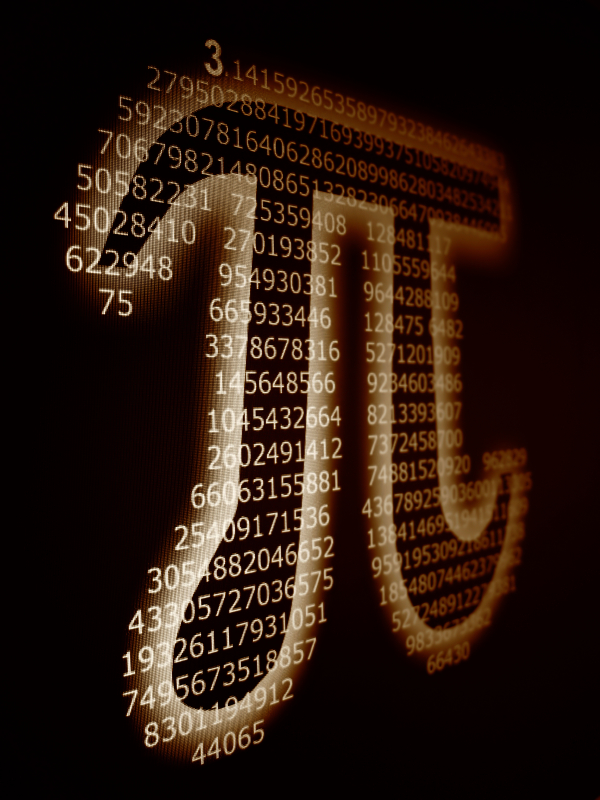## Mathematical Circles: Quadrant IIn the late 1960s and early 1970s, Howard Eves (1911-2004), professor of mathematics at University of Maine, wrote a series of books entitled "In Mathematical Circles." He used the division of a circle into 360 degrees to write 360 short essays exposing the variegated beauties, history, people, humor, and applications of mathematics. On this page, James Nickel will follow the same structure with the goal of unveiling the vistas and power of mathematics as seen through Biblical Christian eyes.

###### Degrees: 1 to 40
• 1: Whither Mathematics Education in the 21st Century?
• 2: Of Stones, Summation, and Academic Sterility (A Biblical Christian world view of the Calculus)
• 3: Quotable Quotes in Mathematics
• 4: Erudite musings from the pen of Stanley L. Jaki (1924-2009)
• 5: The wonders of the Fibonacci Sequence
• 6: Math Books: reviews
• 7: Mathematics Glossary
• 8: The Incarnation and Modern Science
• 9: Clocks: The Hertitage of the Christian Faith
• 10: Saxon Mathematics Program - An Analysis
• 11: The Mathematics textbooks authored by Harold R. Jacobs - An Analysis
• 12: Behind The Bible Code
• 13: The Landscape of Mathematics
• 14: Note on the Study of Mathematics by Alfred North Whitehead (1861-1947)
• 15: Golden (an ode to the Fibonacci Sequence), by Benjamin Moon
• 16: Logos, Rationality, and the Development of Scientific Law
• 17: Why Learn Math?
• 18: Missing Pieces in Math Education
• 19: Where Mathematics Fits in God's World - PowerPoint Slide Show
• 20: Seeing God in Creation through Mathematics - Powerpoint Slide Show
• 21: Algebra: The Language of Science - Powerpoint Slide Show
• 22: Mathematics: Questions, Answers, and Wonder - PowerPoint Slide Show
• 23: A short list of recommended mathematics books (for any school library or interested reader)
• 24: Multiplying with Napier's Bones - PowerPoint Slide Show
• 25: The Golden Rectangle - PowerPoint Slide Show
• 26: Circular Functions (Trigonometry) - PowerPoint Slide Show
• 27: A Biblical Christian Interpretation of Mathematics (ca. 1984)
• 28: Zeno's Paradox - PowerPoint Slide Show
• 29: A short list of arithmetic resources
• 30: Readings in Science
• 31: Baloney Detection: The Right Use of Logic and Investigative Procedure - PowerPoint Slide Show
• 32: Mathematics: The Locus of Reality - PowerPoint Slide Show
• 33: Mathematics: The Utility of Beauty
• 34: Algebra and Number Systems: A Stunning Connection
• 35: Platonic Solids: Why Only Five?
• 36: The Number of Primes: Limitless
• 37: Proof that the square root of 2 is irrational
• 38: Dominoes and Counting
• 39: Let it Snow
• 40: Dr. Julia, meet Dr. Mandelbrot
• ###### Degrees: 41 to 90
• 41: A Case for Calculus
• 42: Keeping Secrets
• 43: Fractions a Plenty
• 44: Algebra: What's It All About?
• 45: The Power of the Continuum
• 46: Euler's Crown Jewel
• 47: How to Forget the Multiplication Table
• 48: Count the Number of Legs and Divide by 4
• 49: Divisibility Rules
• 50: What is Energy?
• 51: Mathematics, Physics, and the Rainbow
• 52: A beautiful proof showing that if a postive integer is not a perfect square then its square root is irrational
• 53: A proof of The Fundamental Theorem of Arithmetic
• 54: Counting, Teaching, and the Trinity: Changing Perspectives
• 55: Whither Mathematics Education in the 21st Century? - Powerpoint Slide Show
• 56: Algebra and the Physics of Motion - Powerpoint Slide Show
• 57: The Third R: Restoring the Rudiments
• 58: Why does i² = -1?
• 59: A proof that e, the base of the natural logarithms, is irrational.
• 60: Finding the Derivative of the Base of the Natural Logarithms function
• 61: Finding the Derivative of the Base of the Natural Logarithms function - Powerpoint Slide Show
• 62: Finding the Derivative of the Sine Function
• 63: Finding the Derivative of the Sine Function - Powerpoint Slide Show
• 64: The Mathematics and Physics of Projectile Motion
• 65: The Proto-Trigonometry of the Pyramids
• 66: Why Radians?
• 67: Mathematics and the Wonders of Creation - Powerpoint Slide Show
• 68: Mathematics and Language
• 69: Epistemology and Mathematical Reasoning
• 70: Functions and Mathematics
• 71: Logarithms and Large Numbers
• 72: The Beauty of Symmetry
• 73: Why Can We Count?
• 74: Mathematics and Uncertainty
• 75: Mathematics and Statistics
• 76: The Nature of Mathematics
• 77: Arithmetic Sequences
• 78: Geometric Sequences
• 79: Power Sequences
• 80: Recursion and the Fibonacci Sequence
• 81: Interpolation, Extrapolation, and the Limits of Science
• 82: What is a Googol?
• 83: Conic Sections: Amazing Unity in Diversity
• 84: Euler's Crown Jewel (An Ode to Euler) - Powerpoint Slide Show
• 85: High School Math Textbooks and Anciliary Material (Recommendations) - Powerpoint Slide Show
• 86: Girls, Time, and Money
• 87: Counting, Infinity, and the Foundation of Knowledge
• 88: Ancient Greek Science and the Rise of Modern Science
• 89: From the Right Triangle to Wave Motion
• 90: A Tribute to Stanley L. Jaki (1924-2009)
• Mathematical Circles II